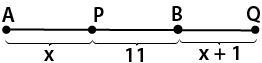Lista de exercícios do ensino médio para impressão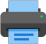Select all.
print selected exercises
Find the lateral area, the total area and the volume of a circular cone that is circumscribed to a sphere of radius $\,r\,$ and whose axial cut is an equilateral triangle.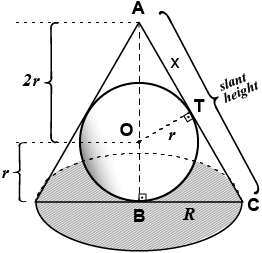answer: $\,S_{\text lat}\,=\,6\,\pi\,r^2\,$; $\,S_{\text total}\,=\,9\,\pi\,r^2\,$; $\,V_{\text olume}\,=\,3\,\pi\,r^3\,$
×
In the following picture:
$\,\overline{PP'}\,$ is the diameter of the sphere whose center is $\,O\,$, $\;M\,$ é is the center of a intersection with a plane perpendicular to $\,\overline{PP'}\,$. Also the measures are $\,\overline{AP}\,=\,6\,cm\;$ and $\,\overline{AP'}\,=\,8\,cm\;$. Calculate the area of the cicle whose center is $\,M\,$.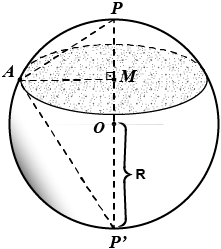answer: The area is $\,\frac{\,576\,\pi\;}{\;25\;}\;cm^2$
×
In the picture the line r is parallel to the line s . Find the value of $\,\hat{\,\alpha\,}\,$.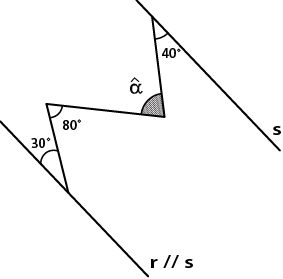×
Knowing that in the picture r // s, the value of $\;\hat{\;x\;}\;$ is:a)
90°
b)
100°
c)
110°
d)
120°
e)
None of the above.

×
Given the following picture, find the value of $\;\hat{\;x\;}\;$ :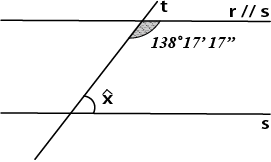×
In the given picture, if r // s , we can say that the value of $\,\hat{\,\alpha\,}\,$ is: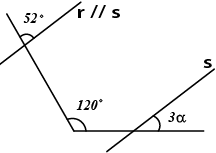a)
90°
b)
100°
c)
110°
d)
120°
e)
22°40'

×
Plot in the following xOy plane the points A(4; 3) , B(-2; 5) , C(-4; -2) , D(3; -4) , E(2; 0) , F(0; -3) , G$(\frac{3}{2};\;\frac{5}{2})\;$ e H($-\frac{1}{2}$; -4).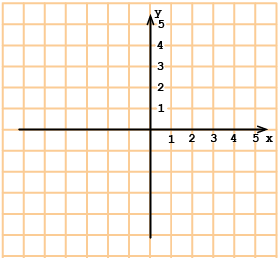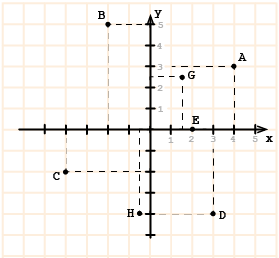×
The lantern shown in the picture is suspended by two strings perpendicular to each other. Knowing the length of each string is 1/2 and 6/5 , respectively, what is the distance between the lantern and the ceiling?
a)
1,69
b)
1,3
c)
0,6
d)
1/2
e)
6/13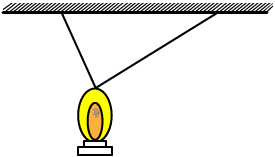×
In the picture below, ABCD is a rectangle, $\,\overline{AB}\,=\,4\,$, $\,\overline{BC}\,=\,1\;$ and $\,\overline{DE}\,=\,\overline{EF}\,=\,\overline{FC}\;$. The length of $\,\overline{BG}\,$ is:
a)
$\,\dfrac{\sqrt{5}}{4}\,$
b)
$\,\dfrac{5}{2}\,$
c)
$\,\dfrac{9}{4}\,$
d)
$\,\dfrac{11}{4}\,$
e)
$\,\dfrac{5}{\sqrt{2}}\,$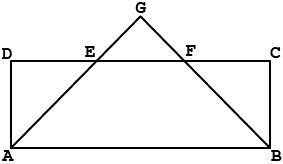×
In the picture below, ABFG and BCDE are squares and their each one sides measures, respectively, a and b. Knowing that $\;\overline{AG}\,=\,\overline{CD}\,+\,2\;\,$ and the perimeter of the triangle ACG is 12, we can assure that a e b are both inside the interval:
a)
]1; 5[
b)
]0; 4[
c)
]2; 6[
d)
]3; 7[
e)
]4; 8[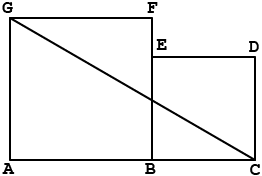×
Knowing that in the right triangle ABC shown in the picture b = 1 and c = 2, find x.
a)
$\,\sqrt{2}\,$
b)
$\,\dfrac{3}{2}\,$
c)
$\,\dfrac{3\sqrt{2}}{2}\,$
d)
$\,\dfrac{2}{3}\,$
e)
$\,\dfrac{2\sqrt{2}}{3}\,$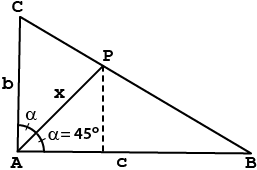×
(FUVEST - 1977)
Given that:
$\,\overline{MP}\;\bot\;s\,$;$\;\overline{MQ}\;\bot\;t\,$;$\;\overline{MQ}\;\bot\;\overline{PQ}\,$;$\;\overline{MP}\,=\,6$
The length of $\,\overline{PQ}\,$ é is:
a)
$\,3\sqrt{3}\,$
b)
$\,3\,$
c)
$\,6\sqrt{3}\,$
d)
$\,4\sqrt{3}\,$
e)
$\,2\sqrt{3}\,$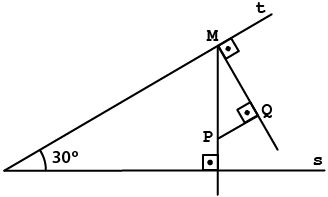×
The picture shows the rhombus ABCD and the point A is the center of the circle that has a 4 cm length radius. Find the area of the rhombus in square centimeters.
a)
$\,4\sqrt{3}\,$
b)
$\,8\,$
c)
$\,12\,$
d)
$\,8\sqrt{3}\,$
e)
$\,12\sqrt{3}\,$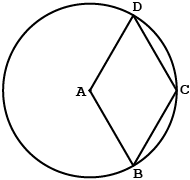×
(FESP - 1991) An equilateral triangle ABC is inscriibed in a circle whose radius is 6 cm length. The triangle is intercepted by a diameter MN of the circle, forming a trapezoid, as shown in the picture below. We can say that the quotient of the triangle ABC area divided by the trapezoid APQC area is:
a)
$\,\dfrac{5}{4}\,$
b)
$\,\dfrac{9}{5}\,$
c)
$\,\dfrac{9}{8}\,$
d)
$\,\dfrac{9}{4}\,$
e)
$\,\dfrac{8}{5}\,$×
AB is the diameter of the circle whose center is O and the triangle ABC is inscribed in. The quotient $\,\dfrac{s}{S}\,$ where the area $\,s\,$ of the triangle ACO is divided by the area $\,S\,$ of the triangle COB is:
a)
$\,\dfrac{5}{4}\,$
b)
$\,\dfrac{4}{3}\,$
c)
$\,\dfrac{3}{4}\,$
d)
$\,1\,$
e)
$\,\dfrac{\sqrt{3}}{2}\,$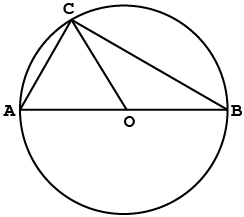×
The circle as shown in the picture below, with center P and radius 2, is tangent to three sides of the rectangle ABCD. Given that the total area of the rectangle is 32, find the distance between the point P and the diagonal AC.
a)
$\,2\dfrac{\sqrt{5}}{5}\,$
b)
$\,\dfrac{\sqrt{5}}{2}\,$
c)
$\,\dfrac{\sqrt{5}}{5}\,$
d)
$\,2\sqrt{5}\,$
e)
$\,3\dfrac{\sqrt{5}}{5}\,$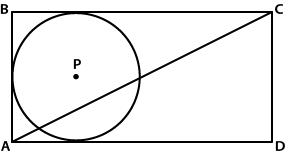×
Knowing that O is the center of the circle, find x in the following cases:
a)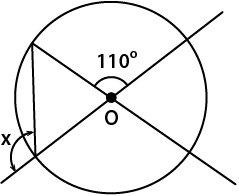b)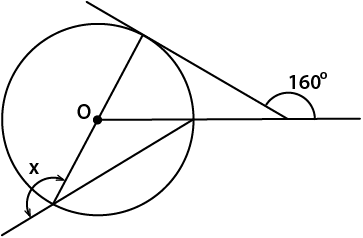×
The circles in the picture are externally tangent. The distance between the centers $\,\overline{OC}\,$ is 28 cm and the difference between their radii is 8 cm. Find the length of each radius.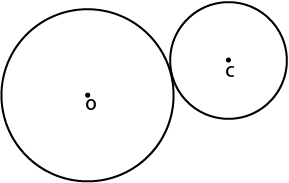answer: 18 cm and 10 cm
×
Find x in the following cases:
a) s is perpendicular to $\;\overline{AB}\,$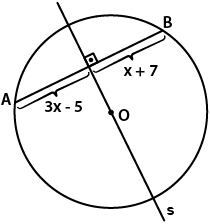b) $\,\overline{PA}\,$ and $\,\overline{PB}\,$ are tangent segments to the circle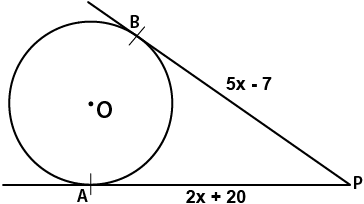×
The radius of the circle C in the picture is 16 cm and the point P is located 7 cm far from the center O.
What is the distance between P and the circunference of the circle C?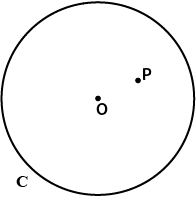×
What is the radius of the following circle which center is O,
given that:
AB = 3x - 3 and
OA = x + 3.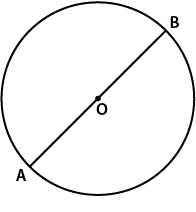×
The length of the arc $\,\stackrel \frown{AB}\,$, as in the picture, is 22 cm and O is the center of the circle. The perimeter of the circle (circumference) is: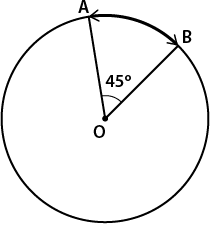a)
990 cm
b)
67 cm
c)
176 cm
d)
88 cm
e)

×
M is the midpoint of AB. Find $\,\overline{AB}\,$ length.
a)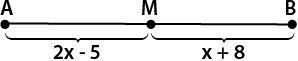b)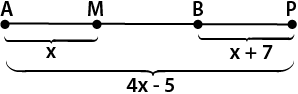×
The length of AB is 17 cm. Find x in the following cases:
a)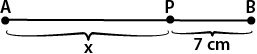b)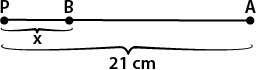c)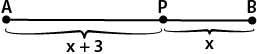d)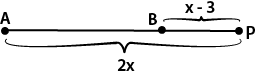a)
10 cm
b)
4 cm
c)
7 cm
d)
14 cm

×
With M being the midpoint of $\,\overline{AB}\,$, find x:
a)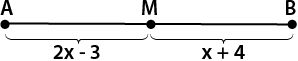b)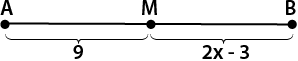×
In the cases a) and b), knowing that AB = 31, what is the length of PQ?
a)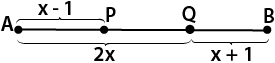b)